# DataWeave reduce function: How to loop through and transform an Array into a different type

10 min read | Last updated on Dec 2022

We would like to thank MuleSoft Ambassador Joshua Erney for their contribution to this developer tutorial.

The `reduce` function is about as close as we get to a general-purpose looping tool in DataWeave. It can be used to transform an Array into any other type. It can be used to perform the task of `map`, `filter`, `distinctBy`, `groupBy`, and other functions that take in Arrays. If you come from a different development background, this is something similar to a `while` or `do while`.

You can try all of these examples with the DataWeave Playground. To learn more about it, check out this tutorial.

## Prerequisites

While not required to follow this tutorial, a good understanding of the basic DataWeave concepts would be preferred. You can check out these other tutorials if you feel a bit lost with some concepts:

## Syntax

The `reduce` function takes two parameters: an Array and a lambda.

With this signature, we can start to see what it means for `reduce` to be general-purpose. The lambda can return anything at all: an Array, Object, String, etc. The type returned from the lambda (`R`) is the same type that is returned from the `reduce` call. The lambda function is defined like this:

It takes in two parameters and returns one. The first parameter (`T`) represents a particular item from the inbound Array. The second parameter (`R`), however, does not represent the current index. The second parameter represents the present value of the accumulator. What’s the accumulator? The accumulator is what stores what is potentially returned from `reduce`. The job of the lambda is to return a new accumulator for each iteration. After the last iteration, the accumulator is what finally gets returned from `reduce`.

## Prefix Notation

This function is not commonly used with the prefix notation because of its complexity. But here you can find an example to sum all of the numbers in an Array. It gives a small glimpse of what `reduce` can be used for.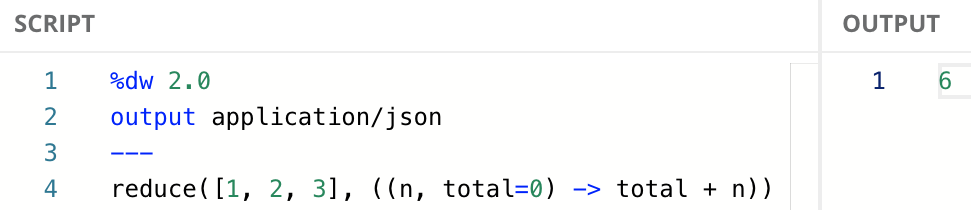## Infix Notation

This is the most used notation for this function because it makes it easier to read and understand.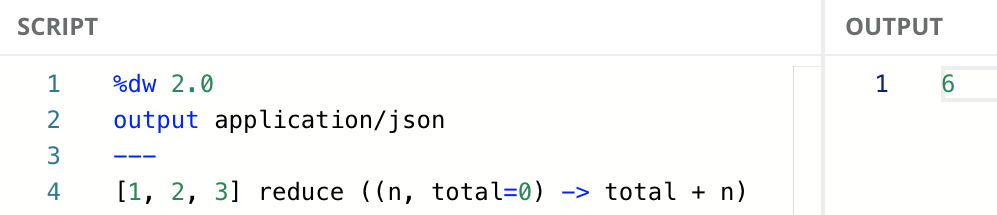You don’t always need to assign an initial value to the accumulator in the lambda (`total=0`). If the initial value for the accumulator is not set, the accumulator is set to the first element of the input Array. For example, `((n, total) -> total + n)`.

## Dollar-sign Syntax

You don’t always need to include the whole explicit lambda expression as the second argument. You can use the dollar-sign syntax to reference the two arguments that are passed to the lambda (i.e., `n` and `total`). `\$` represents the item (`n`), while `\$\$` represents the accumulator (`total`).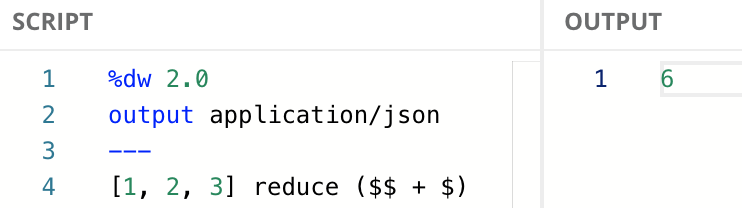You won’t be able to specify a different accumulator type with this syntax. You’ll have to use the explicit lambda in order to do that.

## Step-by-step Process

To illustrate this concept more clearly, let’s take the last example step-by-step and see what’s happening. For the first iteration, `reduce` does something special. If a default value is not declared for the accumulator, it uses the first item of the input Array and passes the second item of the input. So for the first iteration, the lambda is called like this:

Situation: First iteration, no default value provided

 Value (`n` or `\$`) `2` Accumulator (`total` or `\$\$`) `1` Lambda `((2, 1) -> 1 + 2)`

Which returns `3`. We refer to this value as the accumulator. It is used to accumulate the result as `reduce` iterates through the input Array. `reduce` then moves on to the next iteration, which uses the third and final item in the Array. The lambda is called with the current value of the iteration, plus whatever the current value of the accumulator is.

Situation: Second iteration

 Value (`n` or `\$`) `3` Accumulator (`total` or `\$\$`) `3` Lambda `((3, 3) -> 3 + 3)`

Which returns `6`. Notice the first value is `3`, the last value of the input Array, and the second value is `3` as well because that was the previous value of the accumulator. Since this is the last value from the input Array, `reduce` returns the accumulator, which is `6`.

If you’re familiar with the `log` function, you can use the following code to see the iterations and their values in the console:
`[1, 2, 3] reduce (log("res",(log("value", \$\$) + log("acc", \$))))`

## Transform an Array to an Object

The accumulator for `reduce` could be any type. We’re not limited to accumulating values in a scalar like a Number or String. We could accumulate into a collection like an Array if we wanted, or even an Object. In fact, transforming from an Array to an Object is a very common task for `reduce`. Let’s see how to do that with an example.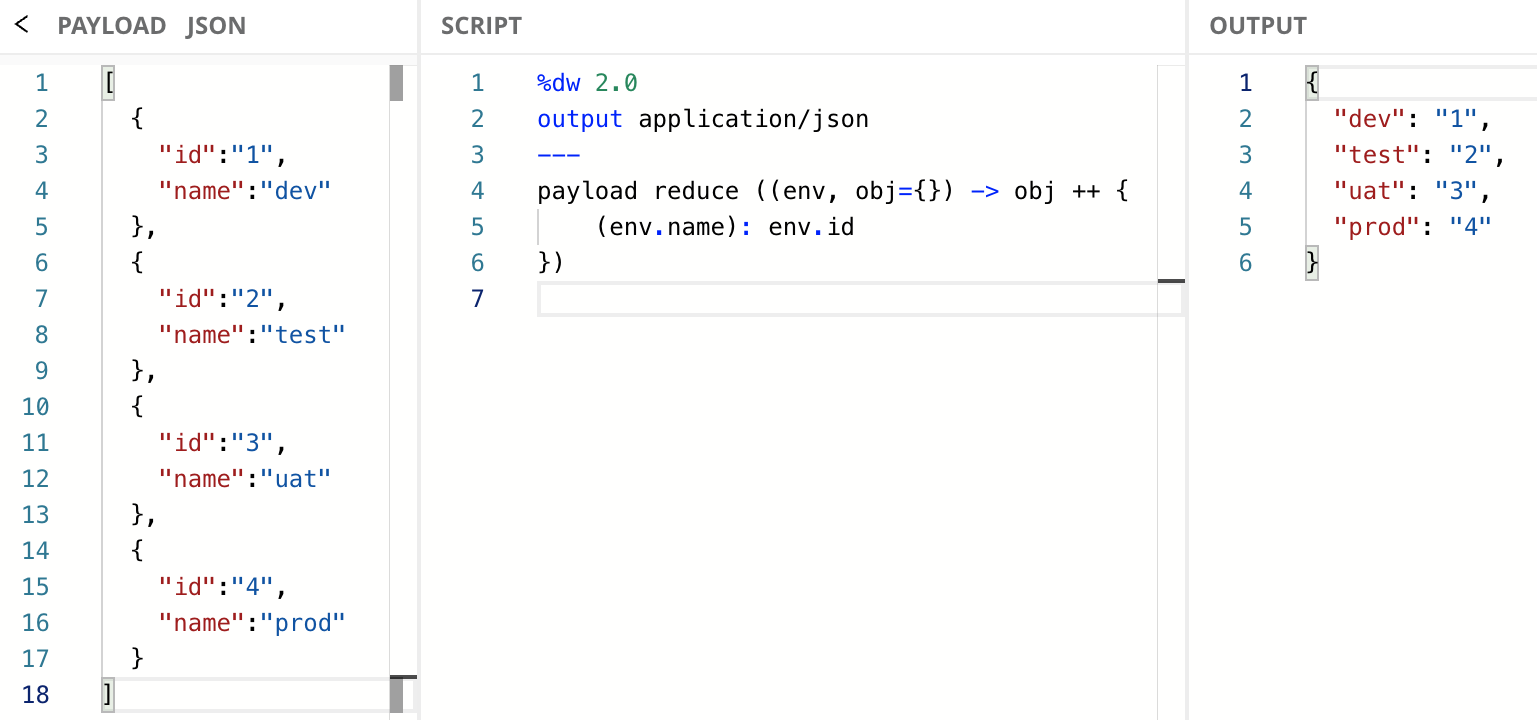You need to surround the `env.name` field in parentheses to correctly reference the value of the environment name when using it as a Key. If you don’t do this, you will receive an error.

If you’re not familiar with the `++` function, it can be used with two Objects to concatenate them into a new Object. All the different ways to use `++` are out of scope for this tutorial, but you can learn more about it in the documentation or this developer tutorial.

## Use Conditionals to Transform the Output

You can also use conditional statements like `if/else` or `match/case` to transform the data into a different type. In this next example, we transform an input Array into a Number. Our `reduce` checks if the `count` field is `true` and adds the `value` into the accumulator (`acc`). If `count` is not true, then it adds a `0`. We end up with the total count of the numbers that had `count=true`.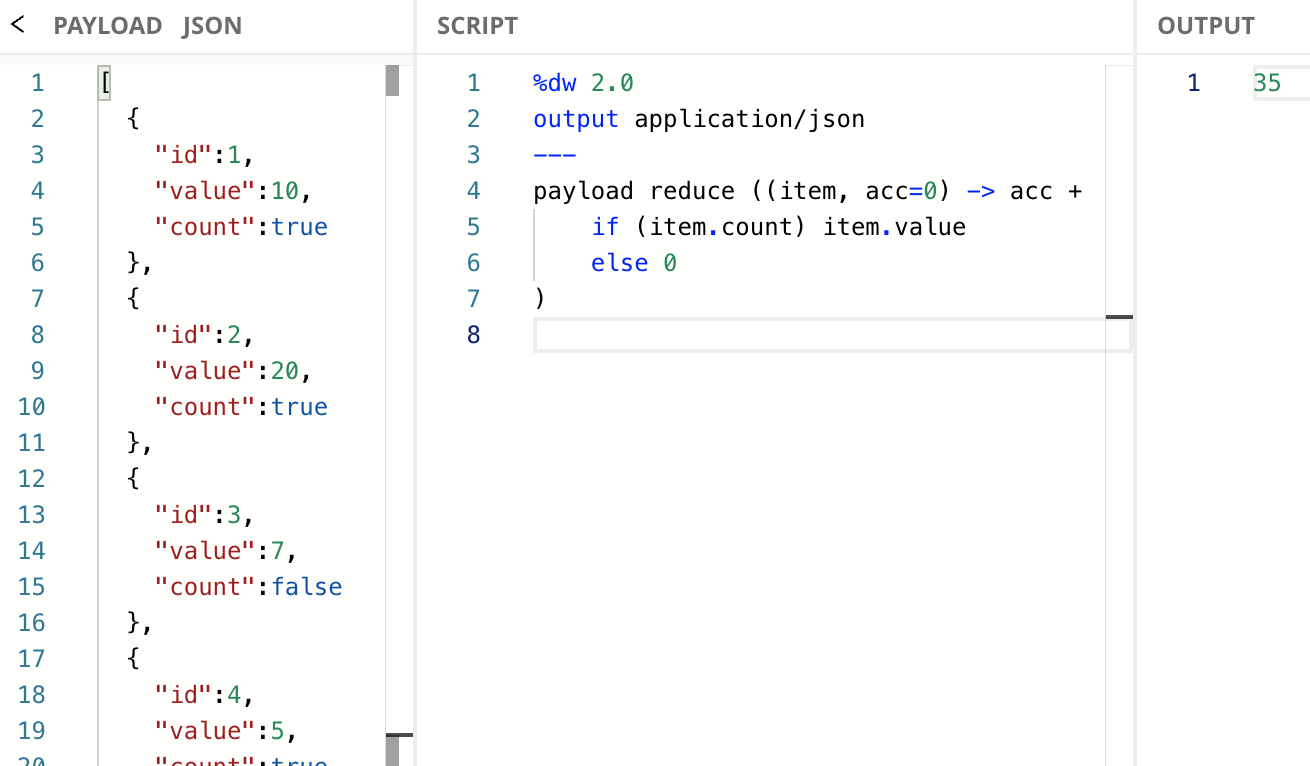## Next Steps

In this tutorial, you learned how to use `reduce` to transform an Array into any other type. It can be used to perform the task of `map`, `filter`, `distinctBy`, `groupBy`, and other functions that take in Arrays. If you come from a different development background, this is something similar to a `while` or `do while`.

Continue your development journey with the rest of the tutorials to become a master in DataWeave.